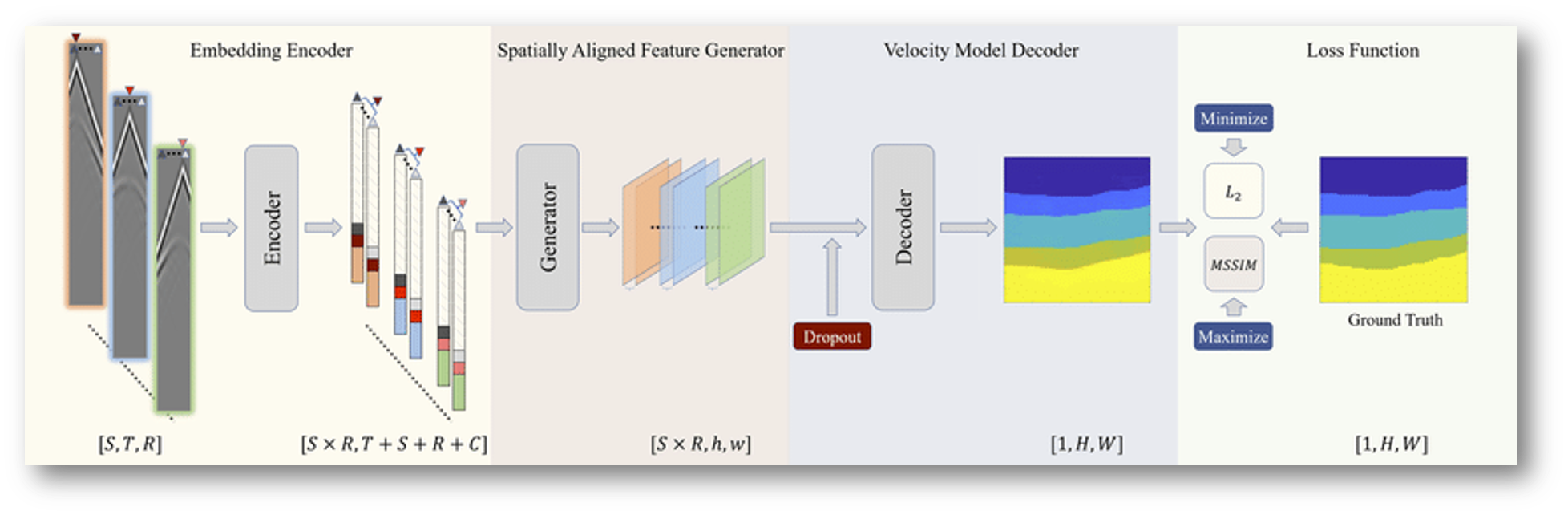# 符号定义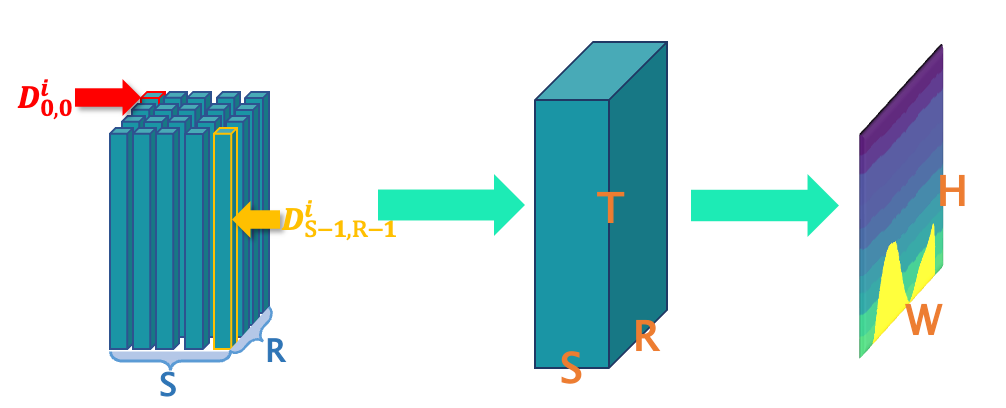• 地震数据$D^i$的大小为$[S,T,R]$，其中$S$$R$$T$分别表示震源个数、接收器个数和时间步长的数量。
• 速度模型$V^i$的大小为$[H, W]$，分别表示速度模型的高度和宽度。

# 网络分析

## 现存问题

$\mathcal{F} (D^i, \theta)\rightarrow V^i$

1. 空间对应性弱，速度模型中的界面与地震数据中的对应模式具有较弱的空间对应关系。
2. 地震数据与速度模型的不确定反射-接收关系。

## 解决方案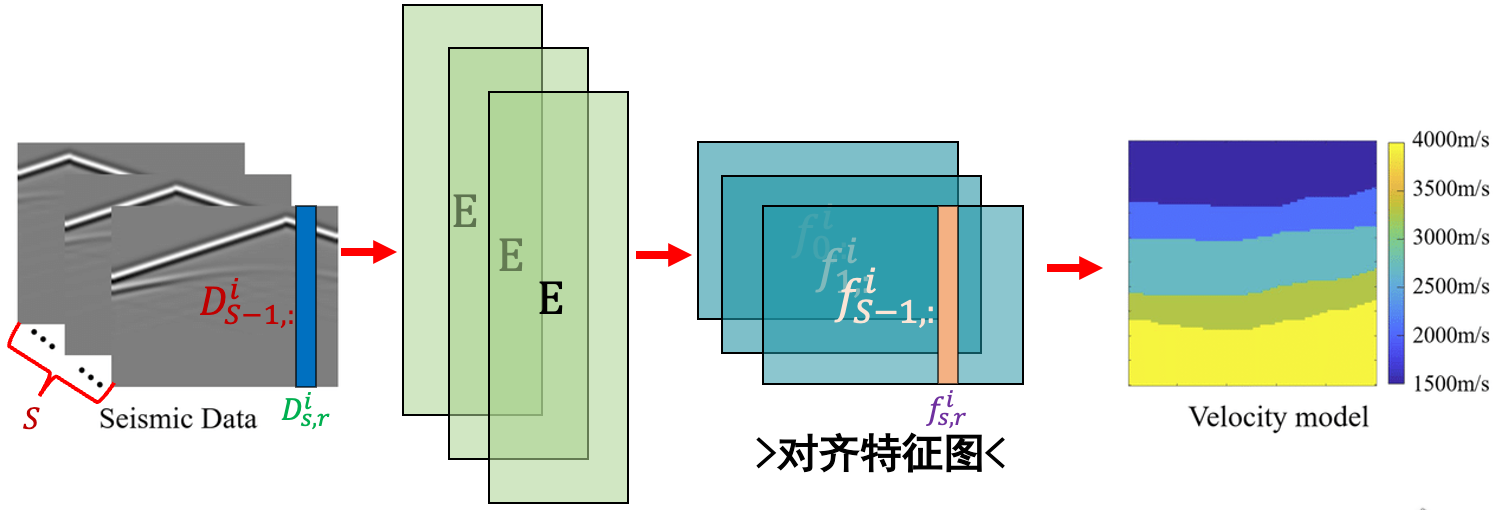$\mathcal{F}:D^i\mathop{\rightarrow}^{\mathcal{N},\mathcal{S},\mathcal{G}}E^i\mathop{\rightarrow}^{\mathcal{F_1}}F_i\mathop{\rightarrow}^{\mathcal{F_2}}V^i$

## 嵌入过程

$E^i_{s,r}=[\mathcal{N}(D^i_{s,r}),\mathcal{S}(D^i_{s,r}),\mathcal{G}(D^i_{s,r})]$

### 邻域信息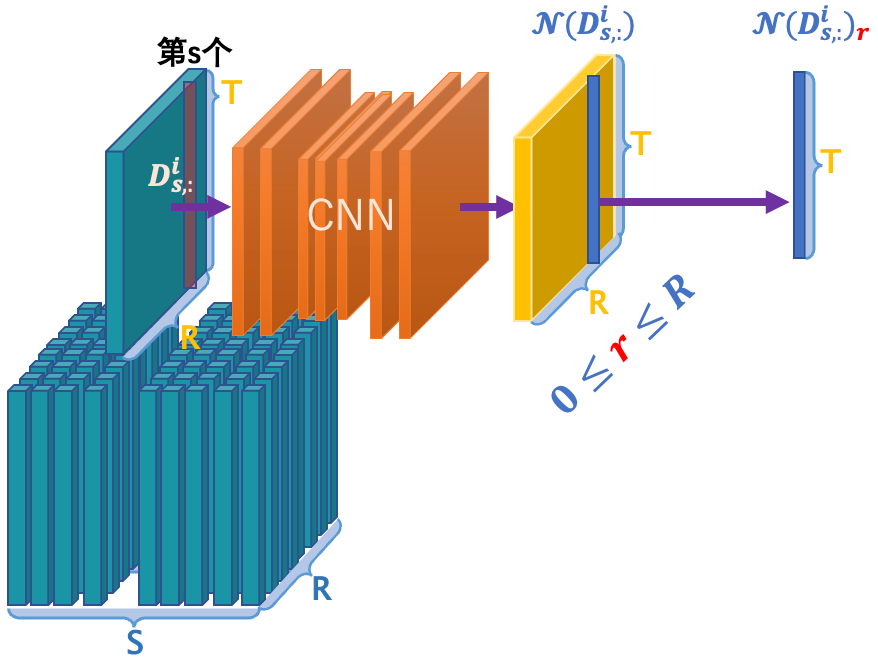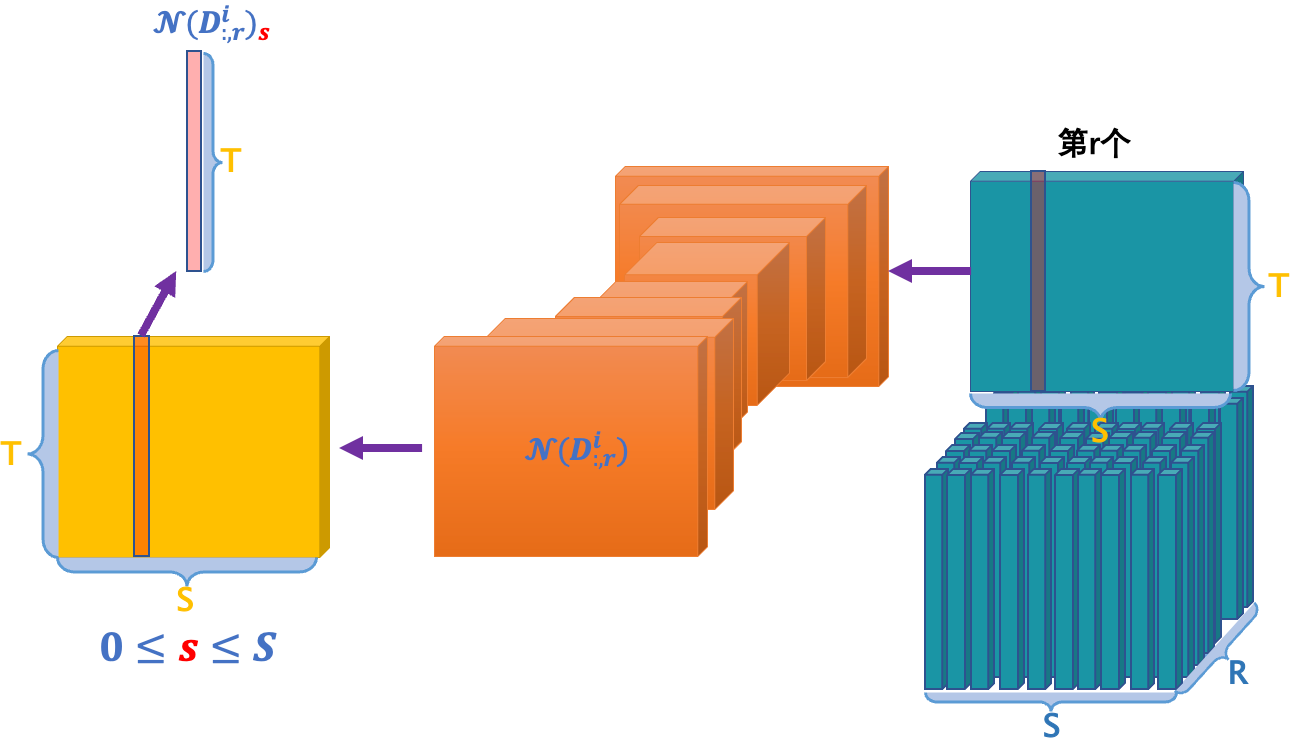$\mathcal{N}(D^i_{s,r})=\frac{1}{2}\mathcal{N(D^i_{s,:})_r}+\frac{1}{2}\mathcal{N(D^i_{:,r})_s}$

### 全局上下文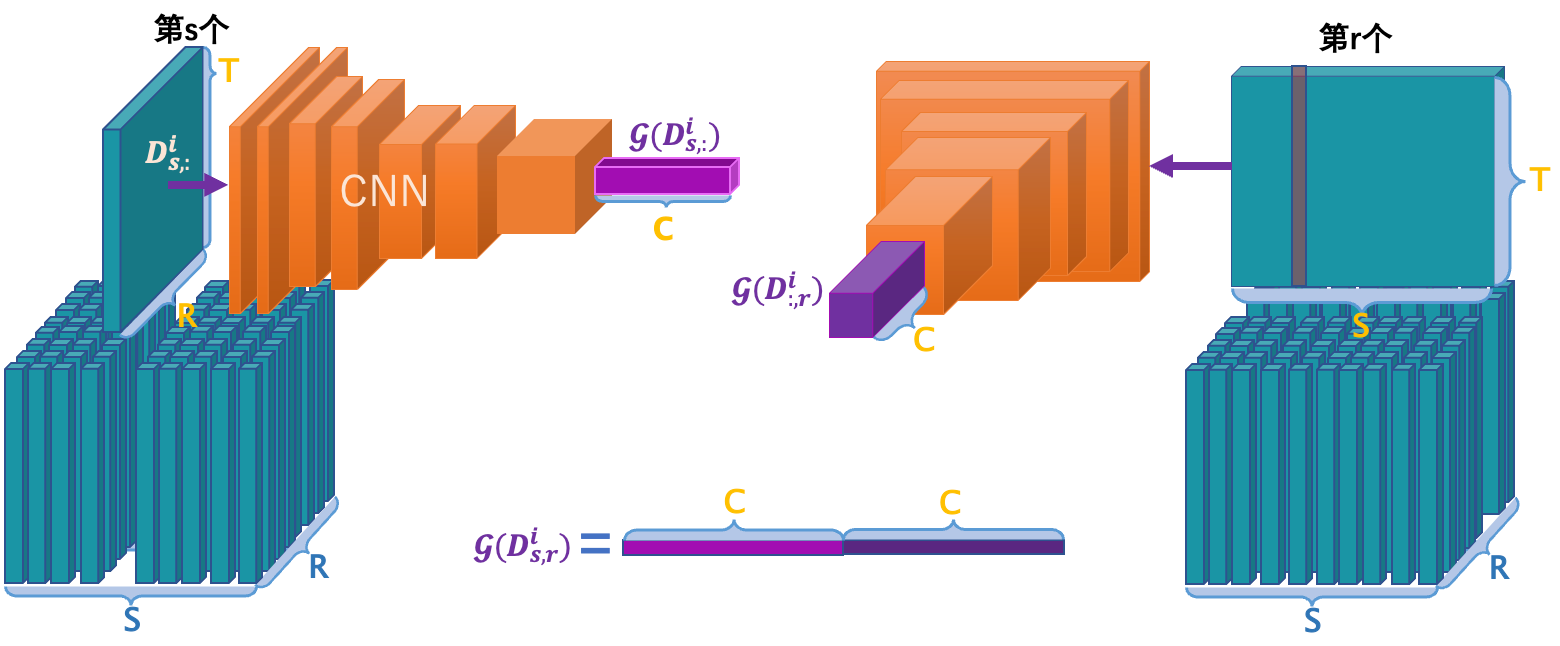## 空间对齐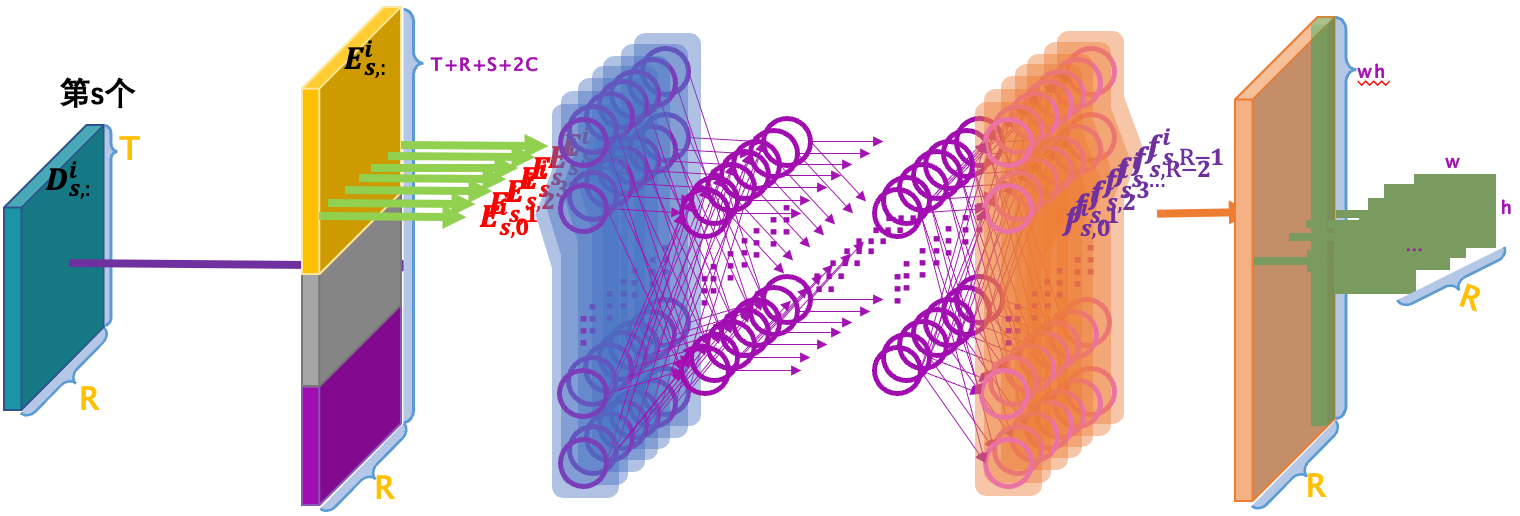## 卷积映射